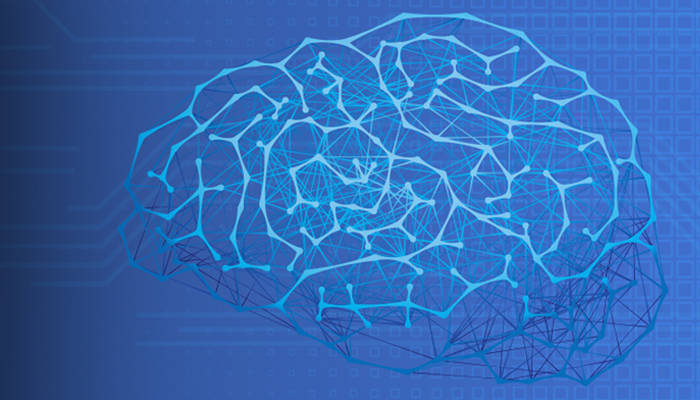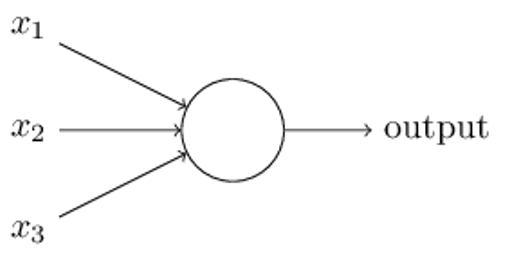﻿ What is a Neural Network? Introduction to Neural Networks Part I – BMC Blogs
Machine Learning & Big Data Blog

# What is a Neural Network? Introduction to Neural Networks Part IbyWe want to explore machine learning on a deeper level by discussing neural networks. We will do that by explaining how you can use Tensor Flow to recognize handwriting. But to do that we first must understand what are neural networks.

We begin our discussion, based upon our knowledge of linear models, and draw some introductory material from this book written by Michael Nielsen. It is recommended by Tensor Flow.

## Intro to Neural Networks

To begin our discussion of how to use TensorFlow to work with neural networks, we first need to discuss what neural networks are.

Think of the linear regression problem we have look at several times here before. We have the concept of a loss function. A neural network hones in on the correct answer to a problem by minimizing the loss function.

Suppose we have this simple linear equation: y = mx + b. This predicts some value of y given values of x.

Predictive models are not always 100% correct. The measure of how incorrect it is is the loss. The goal of machine learning it to take a training set to minimize the loss function. That is true with linear regression, neural networks, and other ML algorithms.

For example, suppose m = 2, x = 3, and b = 2. Then our predicted value of y = 2 * 3 + 2 = 8. But our actual observed value is 10. So the loss is 10 – 8 = 2.

## Perceptrons

In a neural network we have the same basic principle, except the inputs are binary and the outputs are binary. The objects that do the calculations are perceptrons. They adjust themselves to minimize the loss function until the model is very accurate. For example, we can get handwriting analysis to be 99% accurate.

Neural networks are designed to work just like the human brain does. In the case of recognizing handwriting or facial recognition, the brain very quickly makes some decisions. For example, in the case of facial recognition, the brain might start with “It is female or male? Is it black or white? Is it old or young? Is there a scar?” and so forth.

Michael Nielsen lays this out in his book like the diagram below. All of these inputs (x1, x2, x3) are fed into a perceptron. That then makes a yes or no decision and passes it onto the next perceptron for the next decision. This process repeats until the final perceptron. At which point we know what the handwriting is or whose face we are looking at.Let’s illustrate with a small example. This topic is complex, so we will present the first concept here and in the next post take it a step further.

As we said, a perceptron is an object that takes binary inputs and outputs a binary output. It uses a weighted sum and a threshold to decide whether the outcome should be yes (1) or no (0).

For example, suppose you want to go to France but only if:

x1 -> The airline ticket is less than \$1,000.
x2 -> Your girlfriend or boyfriend can go with you.

You represent this decision with this simple vector of possible inputs:

(1,0), (0,1), (1,1), and (0,0).

In the first case (1,0) the ticket is > 1,000 and your girlfriend or boyfriend cannot go with you.

You put some weight on each of these two calculations. For example, if you are on a budget and cost is important, give it weight w1=4. And whether your partner can go or not is not as important. So give it a weight of w2=3.

So you have this function for Go to France:

(x1 * w1) + (x2 * w2) = (x1 * 4) + (x2 * 3) > some threshold, b, say, 4.

We move b to the other side and write:

If (x1 * 4) + (x2 * 3) -4 > 0 then Go to France (i.e., perceptron says 1)-

Then feed vectors into the equation. Obviously if the ticket is > \$1,000 and if your girlfriend cannot go (0,0) then you will not make the trip, because

(0 * 3) + (0 * 4) – 4 is obviously < 0.

If the ticket is cheap but you are going alone then go anyway:

(1 * 4) + (0 * 3) – 4 = 0 which is not bigger than 0.

## Handwriting Recognition

Handwriting and facial recognition using neural networks does the same thing, meaning making a series of binary decisions. This is because any image can be broken down into its smallest object, the pixel. In the case of handwriting, like shown below, each pixel is either black (1) or white (meaning empty, or 0).Graphic Source Michael Neilson.

In the next post we will move onto the next concept to master.

### Wikibon: Automate your Big Data pipeline

Learn how data management experts throughout the industry are transforming their Big Data infrastructure for maximum business impact.

These postings are my own and do not necessarily represent BMC's position, strategies, or opinion.

See an error or have a suggestion? Please let us know by emailing blogs@bmc.com.

### About the author#### Walker Rowe

Walker Rowe is an American freelance tech writer and programmer living in Tunisia. He specializes in big data, analytics, and programming languages. Find him on LinkedIn or Upwork.